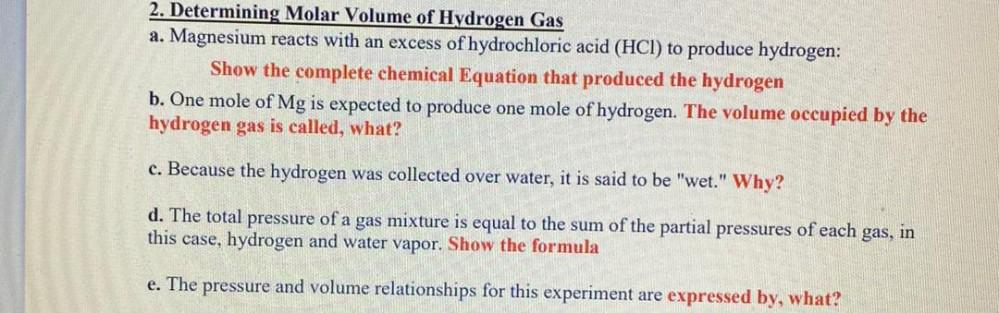Question:

# 2. Determining Molar Volume of Hydrogen Gas a. Magnesium reacts with an excess of hydrochloric acid (HCl) to produce hydrogen: S2. Determining Molar Volume of Hydrogen Gas a. Magnesium reacts with an excess of hydrochloric acid (HCl) to produce hydrogen: Show the complete chemical Equation that produced the hydrogen b. One mole of Mg is expected to produce one mole of hydrogen. The volume occupied by the hydrogen gas is called, what? c. Because the hydrogen was collected over water, it is said to be "wet." Why? d. The total pressure of a gas mixture is equal to the sum of the partial pressures of each gas, in this case, hydrogen and water vapor. Show the formula e. The pressure and volume relationships for this experiment are expressed by, what?